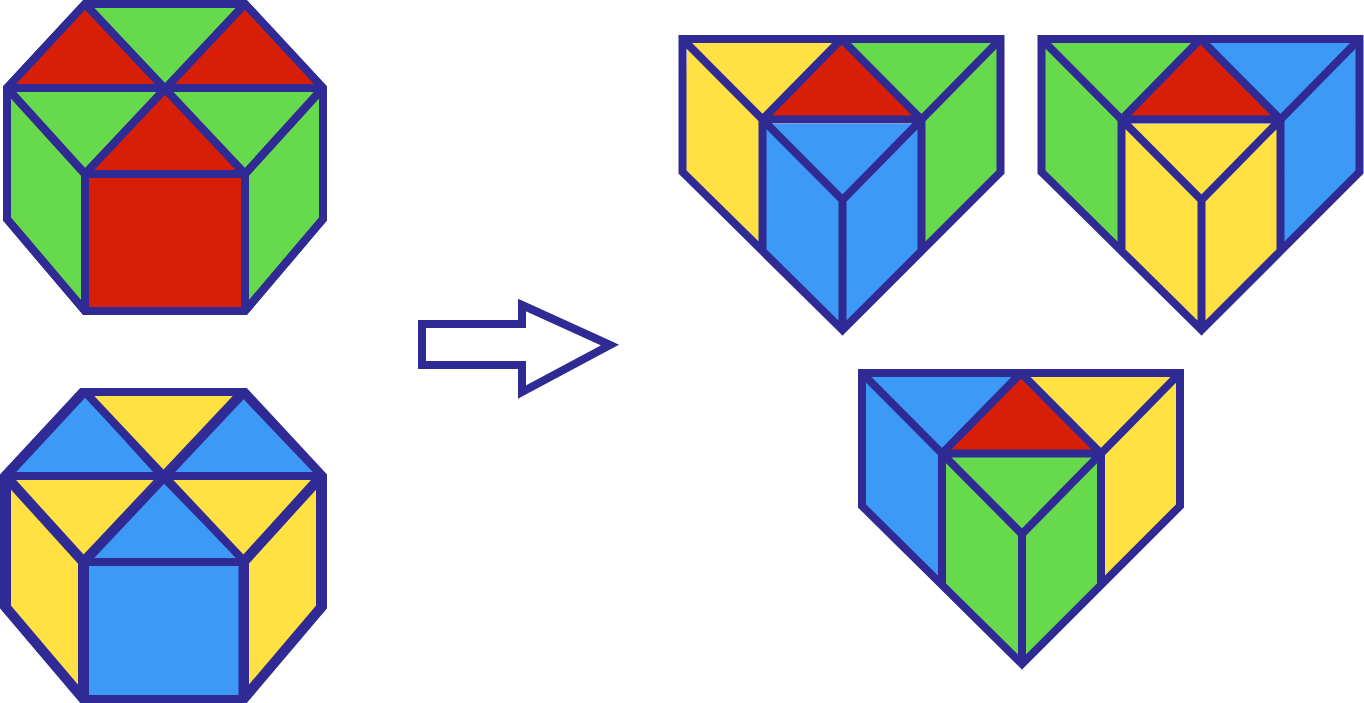# Colorful Prisms

Geometry Level 2Two equivalent regular hexagonal-based prisms are divided and rearranged into 3 equilateral triangular-based prisms, as shown above. After rearrangement, the total surface area of all prisms has increased by 12.5% with the constant height.

If the height of each prism is $\sqrt{3} \text{ cm}$, what is the side length of the regular hexagon base in $\text{cm}$?

×